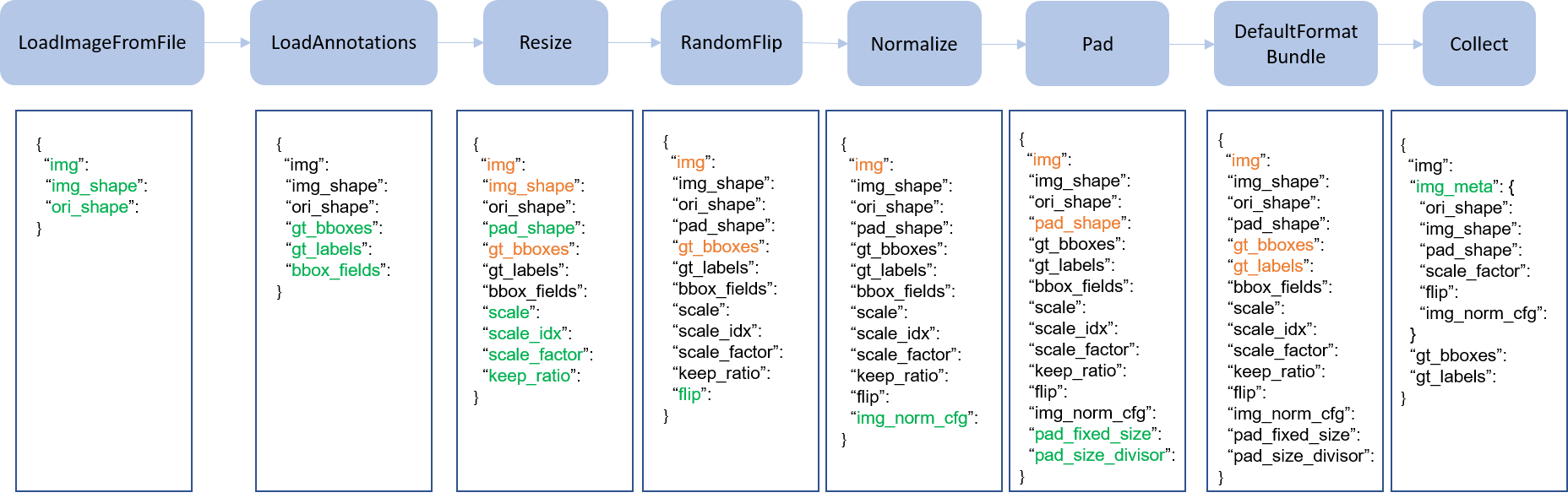Shortcuts

# 数据变换（待更新）¶img_norm_cfg = dict(
mean=[123.675, 116.28, 103.53], std=[58.395, 57.12, 57.375], to_rgb=True)
train_pipeline = [
dict(type='Resize', img_scale=(1333, 800), keep_ratio=True),
dict(type='RandomFlip', flip_ratio=0.5),
dict(type='Normalize', **img_norm_cfg),
dict(type='DefaultFormatBundle'),
dict(type='Collect', keys=['img', 'gt_bboxes', 'gt_labels']),
]
test_pipeline = [
dict(
type='MultiScaleFlipAug',
img_scale=(1333, 800),
flip=False,
transforms=[
dict(type='Resize', keep_ratio=True),
dict(type='RandomFlip'),
dict(type='Normalize', **img_norm_cfg),
dict(type='ImageToTensor', keys=['img']),
dict(type='Collect', keys=['img']),
])
]


© Copyright 2018-2021, OpenMMLab. Revision 92d03df6.

Built with Sphinx using a theme provided by Read the Docs.
Versions
latest
stable
3.x
v2.27.0
v2.26.0
v2.25.3
v2.25.2
v2.25.1
v2.25.0
v2.24.1
v2.24.0
v2.23.0
v2.22.0
v2.21.0
v2.20.0
v2.19.1
v2.19.0
v2.18.1
v2.18.0
v2.17.0
v2.16.0
v2.15.1
v2.15.0
v2.14.0
v2.13.0
dev-3.x
dev